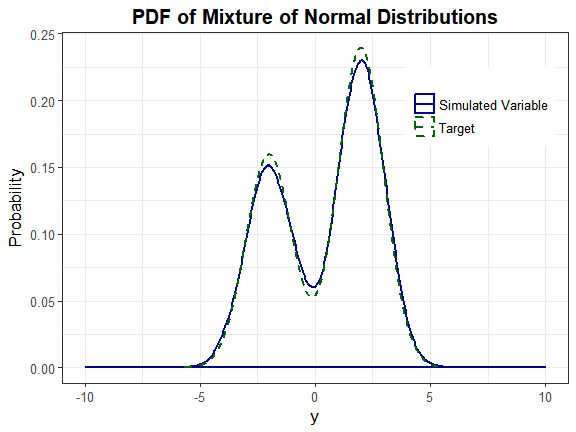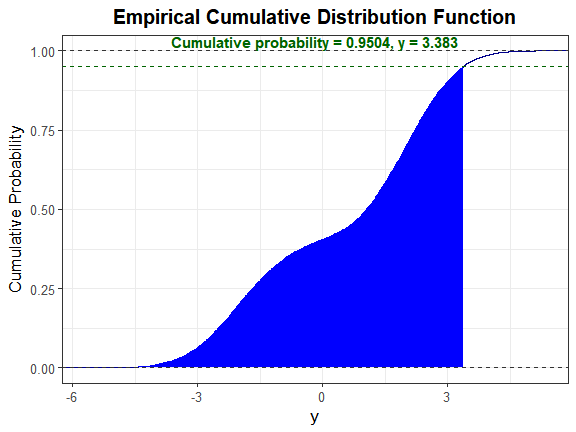# Simulation of Correlated Data with Multiple Variable Types Including Continuous and Count Mixture Distributions

Generate continuous (normal, non-normal, or mixture distributions), binary, ordinal, and count (regular or zero-inflated, Poisson or Negative Binomial) variables with a specified correlation matrix, or one continuous variable with a mixture distribution. This package can be used to simulate data sets that mimic real-world clinical or genetic data sets (i.e., plasmodes, as in Vaughan et al., 2009 ). The methods extend those found in the 'SimMultiCorrData' R package. Standard normal variables with an imposed intermediate correlation matrix are transformed to generate the desired distributions. Continuous variables are simulated using either Fleishman (1978)'s third order or Headrick (2002)'s fifth order polynomial transformation method (the power method transformation, PMT). Non-mixture distributions require the user to specify mean, variance, skewness, standardized kurtosis, and standardized fifth and sixth cumulants. Mixture distributions require these inputs for the component distributions plus the mixing probabilities. Simulation occurs at the component level for continuous mixture distributions. The target correlation matrix is specified in terms of correlations with components of continuous mixture variables. These components are transformed into the desired mixture variables using random multinomial variables based on the mixing probabilities. However, the package provides functions to approximate expected correlations with continuous mixture variables given target correlations with the components. Binary and ordinal variables are simulated using a modification of ordsample() in package 'GenOrd'. Count variables are simulated using the inverse CDF method. There are two simulation pathways which calculate intermediate correlations involving count variables differently. Correlation Method 1 adapts Yahav and Shmueli's 2012 method and performs best with large count variable means and positive correlations or small means and negative correlations. Correlation Method 2 adapts Barbiero and Ferrari's 2015 modification of the 'GenOrd' package and performs best under the opposite scenarios. The optional error loop may be used to improve the accuracy of the final correlation matrix. The package also contains functions to calculate the standardized cumulants of continuous mixture distributions, check parameter inputs, calculate feasible correlation boundaries, and summarize and plot simulated variables.

# SimCorrMix

The goal of SimCorrMix is to generate continuous (normal, non-normal, or mixture distributions), binary, ordinal, and count (Poisson or Negative Binomial, regular or zero-inflated) variables with a specified correlation matrix, or one continuous variable with a mixture distribution. This package can be used to simulate data sets that mimic real-world clinical or genetic data sets (i.e. plasmodes, as in Vaughan et al., 2009, doi:10.1016/j.csda.2008.02.032). The methods extend those found in the SimMultiCorrData package. Standard normal variables with an imposed intermediate correlation matrix are transformed to generate the desired distributions. Continuous variables are simulated using either Fleishman (1978)'s third-order (doi:10.1007/BF02293811) or Headrick (2002)'s fifth-order (doi:10.1016/S0167-9473(02)00072-5) power method transformation (PMT). Non-mixture distributions require the user to specify mean, variance, skewness, standardized kurtosis, and standardized fifth and sixth cumulants. Mixture distributions require these inputs for the component distributions plus the mixing probabilities. Simulation occurs at the component-level for continuous mixture distributions. The target correlation matrix is specified in terms of correlations with components of continuous mixture variables. These components are transformed into the desired mixture variables using random multinomial variables based on the mixing probabilities. However, the package provides functions to determine expected correlations with continuous mixture variables given target correlations with the components. Binary and ordinal variables are simulated using a modification of GenOrd-package's ordsample function. Count variables are simulated using the inverse CDF method. There are two simulation pathways which calculate intermediate correlations involving count variables differently. Correlation Method 1 adapts Yahav and Shmueli's 2012 method (doi:10.1002/asmb.901) and performs best with large count variable means and positive correlations or small means and negative correlations. Correlation Method 2 adapts Barbiero and Ferrari's 2015 modification of the GenOrd package (doi:10.1002/asmb.2072) and performs best under the opposite scenarios. The optional error loop may be used to improve the accuracy of the final correlation matrix. The package also contains functions to calculate the standardized cumulants of continuous mixture distributions, check parameter inputs, calculate feasible correlation boundaries, and plot simulated variables.

There are several vignettes which accompany this package that may help the user understand the simulation and analysis methods.

1. Comparison of Correlation Methods 1 and 2 describes the two simulation pathways that can be followed for generation of correlated data (using `corrvar` and `corrvar2`).

2. Continuous Mixture Distributions demonstrates how to simulate one continuous mixture variable using `contmixvar1` and gives a step-by-step guideline for comparing a simulated distribution to the target distribution.

3. Expected Cumulants and Correlations for Continuous Mixture Variables derives the equations used by the function `calc_mixmoments` to find the mean, standard deviation, skew, standardized kurtosis, and standardized fifth and sixth cumulants for a continuous mixture variable. The vignette also explains how the functions `rho_M1M2` and `rho_M1Y` calculate the expected correlations with continuous mixture variables based on the target correlations with the components.

4. Overall Workflow for Generation of Correlated Data gives a step-by-step guideline to follow with an example containing continuous non-mixture and mixture, ordinal, zero-inflated Poisson, and zero-inflated Negative Binomial variables. It executes both correlated data simulation functions with and without the error loop.

5. Variable Types describes the different types of variables that can be simulated in SimCorrMix, details the algorithm involved in the optional error loop that helps to minimize correlation errors, and explains how the feasible correlation boundaries are calculated for each of the two simulation pathways (using `validcorr` and `validcorr2`).

## Installation instructions

SimCorrMix can be installed using the following code:

## Example

This is a basic example which shows you how to solve a common problem:

Headrick and Kowalchuk's steps (2007, doi:10.1080/10629360600605065) to compare the density of a simulated variable to the theoretical density have been modified to accommodate continuous mixture distributions. The component distributions are Normal(-2, 1) and Normal(2, 1). The mixing proportions are 0.4 and 0.6.

### Step 1: Obtain the standardized cumulants

The values of γ1,  γ2,  γ3, and γ4 are all 0 for normal variables. The mean and standard deviation of the mixture variable are found with `calc_mixmoments`.

### Step 2: Simulate the variable

Note that `calc_mixmoments` returns the standard deviation, not the variance. The simulation functions require variance as the input. First, the parameter inputs are checked with `validpar`.

Look at a summary of the target distribution and compare to a summary of the simulated distribution.

Distribution Mean SD Skew Skurtosis Fifth Sixth
1 0.4 2.2 -0.2885 -1.15402 1.79302 6.17327
Distribution N Mean SD Median Min Max Skew Skurtosis Fifth Sixth
1 10000 0.4 2.19989 1.05078 -5.69433 5.341 -0.2996 -1.15847 1.84723 6.1398

### Step 3: Determine if the constants generate a valid PDF

c0 c1 c2 c3 c4 c5
0 1 0 0 0 0
0 1 0 0 0 0

### Step 4: Select a critical value

Let α = 0.05. Since there are no quantile functions for mixture distributions, determine where the cumulative probability equals 1 − α = 0.95. The boundaries for `uniroot` were determined through trial and error.

### Step 5: Calculate the cumulative probability for the simulated variable up to 1 − α

We will use the function `SimMultiCorrData::sim_cdf_prob` to determine the cumulative probability for Y up to `y_star`. This function is based on Martin Maechler's `ecdf` function [@Stats].

This is approximately equal to the 1 − α value of 0.95, indicating the method provides a good approximation to the actual distribution.

### Step 6: Plot graphsWe can also plot the empirical cdf and show the cumulative probability up to y_star.# SimCorrMix 0.1.1

Updated some citations.

# SimCorrMix 0.1.0

Initial package release.

# Reference manual

install.packages("SimCorrMix")

0.1.1 by Allison Cynthia Fialkowski, a year ago

https://github.com/AFialkowski/SimCorrMix

Browse source code at https://github.com/cran/SimCorrMix

Authors: Allison Cynthia Fialkowski

Documentation:   PDF Manual

GPL-2 license

Imports BB, nleqslv, MASS, mvtnorm, Matrix, VGAM, triangle, ggplot2, grid, stats, utils

Depends on SimMultiCorrData

Suggests knitr, rmarkdown, printr, bookdown, testthat

Depended on by SimRepeat.

See at CRAN